# Magnetism and Matter Questions and Answers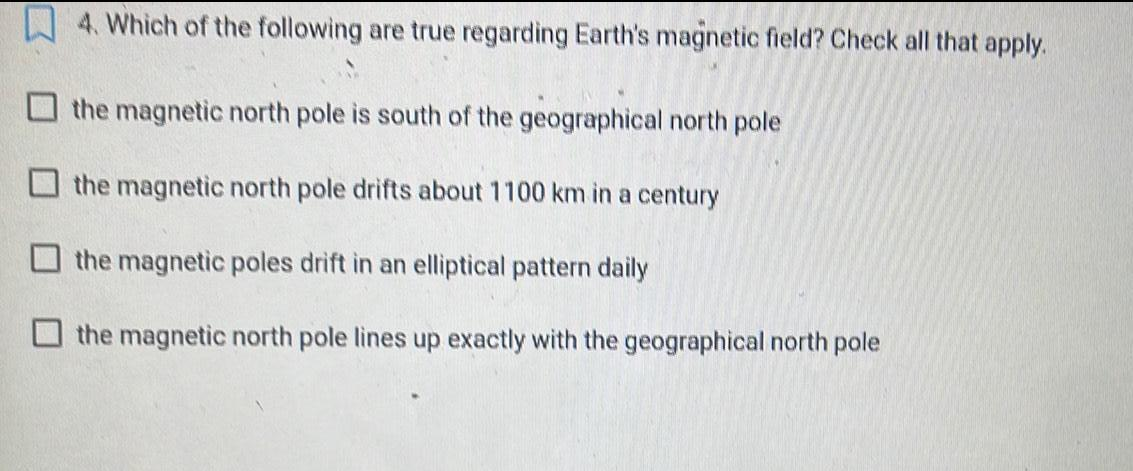Physics
Magnetism and Matter
4. Which of the following are true regarding Earth's magnetic field? Check all that apply. the magnetic north pole is south of the geographical north pole the magnetic north pole drifts about 1100 km in a century the magnetic poles drift in an elliptical pattern daily the magnetic north pole lines up exactly with the geographical north pole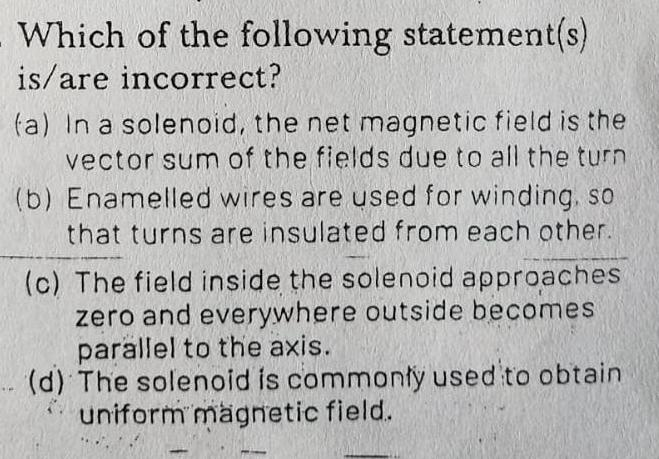Physics
Magnetism and Matter
Which of the following statement(s) is/are incorrect? (a) In a solenoid, the net magnetic field is the vector sum of the fields due to all the turn (b) Enamelled wires are used for winding, so that turns are insulated from each other. (c) The field inside the solenoid approaches zero and everywhere outside becomes parallel to the axis. (d) The solenoid is commonly used to obtain uniform magnetic field.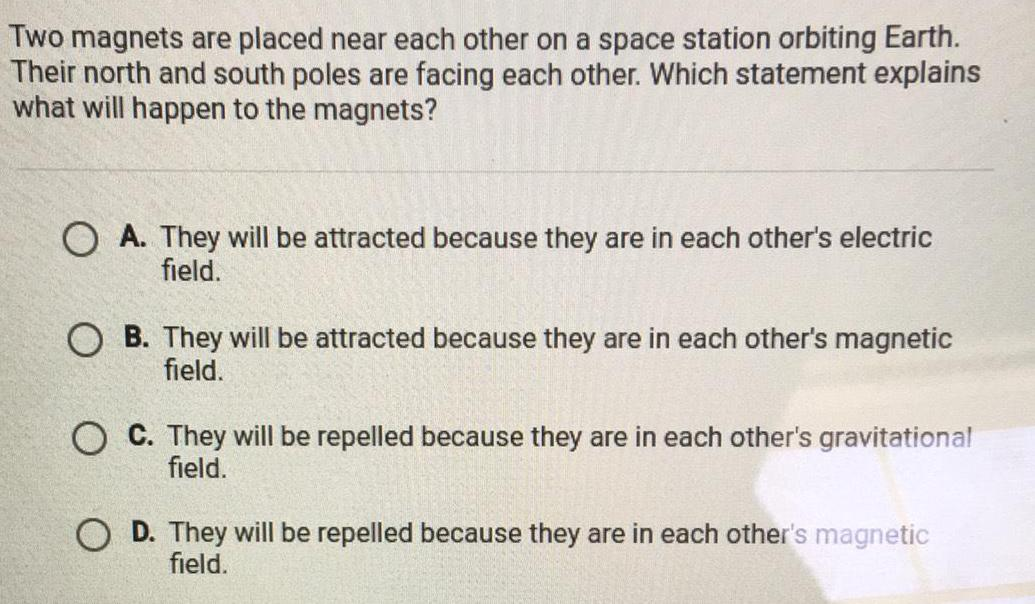Physics
Magnetism and Matter
Two magnets are placed near each other on a space station orbiting Earth. Their north and south poles are facing each other. Which statement explains what will happen to the magnets A. They will be attracted because they are in each other's electric field. B. They will be attracted because they are in each other's magnetic field. C. They will be repelled because they are in each other's gravitational field. D. They will be repelled because they are in each other's magnetic field.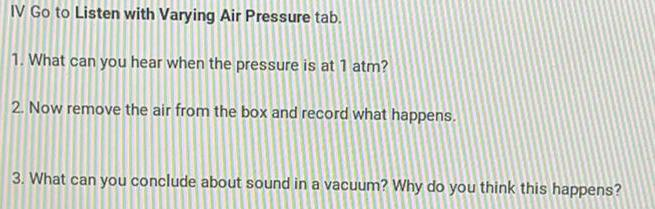Physics
Magnetism and Matter
IV Go to Listen with Varying Air Pressure tab. 1. What can you hear when the pressure is at 1 atm? 2. Now remove the air from the box and record what happens. 3. What can you conclude about sound in a vacuum? Why do you think this happens?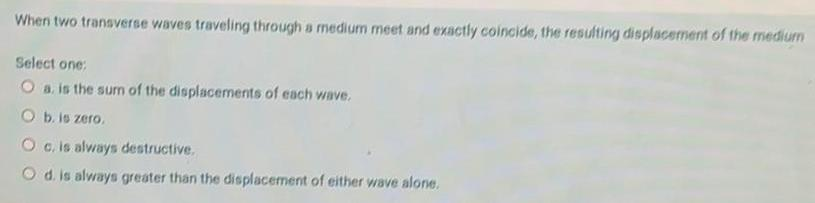Physics
Magnetism and Matter
When two transverse waves traveling through a medium meet and exactly coincide, the resulting displacement of the medium Select one: a. is the sum of the displacements of each wave. b. is zero. c. is always destructive. d. is always greater than the displacement of either wave alone.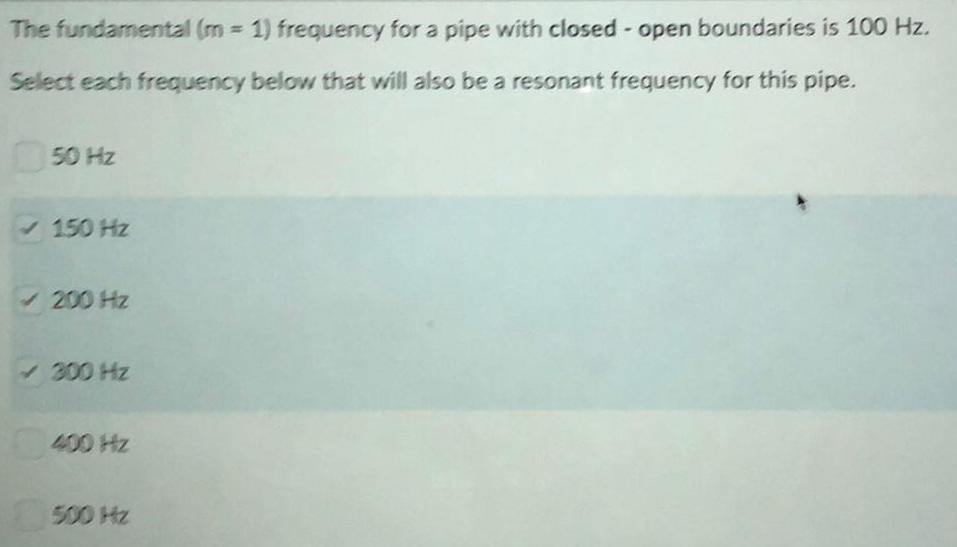Physics
Magnetism and Matter
The fundamental (m= 1) frequency for a pipe with closed - open boundaries is 100 Hz. Select each frequency below that will also be a resonant frequency for this pipe. 50 Hz 150 Hz 200 Hz 300 Hz 400 Hz 500 Hz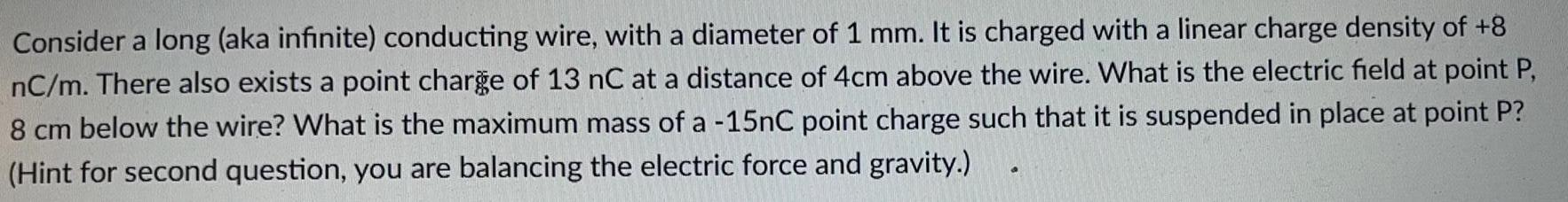Physics
Magnetism and Matter
Consider a long (aka infinite) conducting wire, with a diameter of 1 mm. It is charged with a linear charge density of +8 nC/m. There also exists a point charge of 13 nC at a distance of 4cm above the wire. What is the electric field at point P, 8 cm below the wire? What is the maximum mass of a -15nC point charge such that it is suspended in place at point P? (Hint for second question, you are balancing the electric force and gravity.)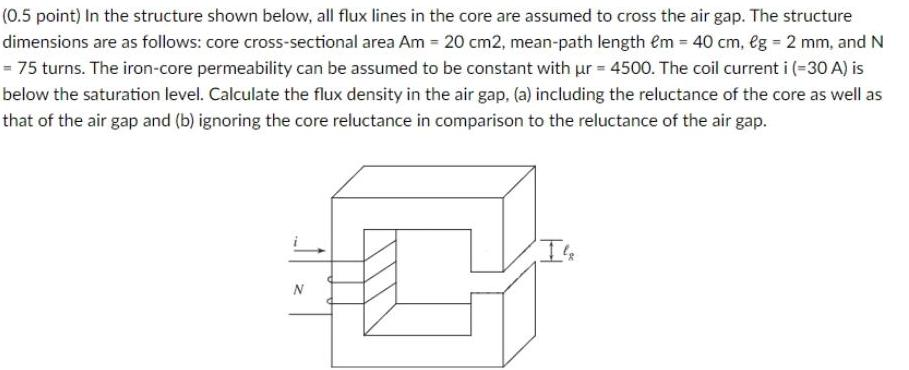Physics
Magnetism and Matter
In the structure shown below, all flux lines in the core are assumed to cross the air gap. The structure dimensions are as follows: core cross-sectional area Am = 20 cm2, mean-path length em = 40 cm, eg = 2 mm, and N = 75 turns. The iron-core permeability can be assumed to be constant with ur = 4500. The coil current i (-30 A) is below the saturation level. Calculate the flux density in the air gap, (a) including the reluctance of the core as well as that of the air gap and (b) ignoring the core reluctance in comparison to the reluctance of the air gap.
1